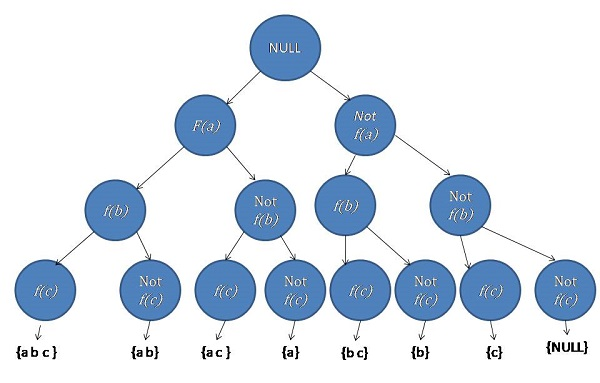# Power Set in Lexicographic order

Power Set in Lexicographic order: Here, we are going to learn to find out the power sets in lexicographic order of a given set of numbers using backtracking.
Submitted by Souvik Saha, on February 04, 2020

Description:

This is a standard interview problem to find out the power sets in lexicographic order of a given set of numbers using backtracking.

Problem statement:

There is a set contains N no. of unsorted characters. You have to find out the power sets in lexicographic order of a given set of numbers and print them.

```    Input:
Test case T
//T no. of line with the value of N and corresponding values.

E.g.
2
4
d c b a

2
f u

1<=T<=100
1<=N<=1000

Output:
Print the power subsets of the set in lexicographic order.
```

Example

```    T=2

N=4
d c b a

Output:
a
a b
a b c
a b c d
a b d
a c
a c d
a d
b
b c
b c d
b d
c
c d
d

N=2
f u

Output:
f
f u
u
```

Explanation with example

Let, there is a Set S having N no. of numbers.

```    S = {X1, X2, X3, ..., Xn}
```

The process to print the subsets of the set is a problem of combination and permutation. To get the result we use the backtracking process.

First, we will have to sort the character and then we will apply our backtracking procedure.

Let, f(i) = function to insert the ith number into a subset.

Here, we take a subset of that set in our consideration and consider two things,

1. An element is a part of that subset (f(i)).
2. An element is not a part of that subset (not f(i)).
```For     N=3
S = {a b c}
```Figure 1: Recursion Tree

Algorithm:

Here we use the vector STL to store the subsets.

```    traverse(arr, n, current_pos,set,subset){
if(Current_pos is greater or equals to the n)Then
return
end if

for i= current_pos to the end of the set
insert the element of arr[i] into subset
insert the subset into the set
traverse(arr,n,i+1,set,subset)
pop the element from subset
end for
}
```

C++ implementation:

```#include <bits/stdc++.h>
using namespace std;

void traverse(char* arr, int n, int pos, vector<vector<char> >& v, vector<char> vi)
{
//if the current position is greater than or equals to n
if (pos >= n)
return;
for (int i = pos; i < n; i++) {
vi.push_back(arr[i]);
v.push_back(vi);
traverse(arr, n, i + 1, v, vi);
vi.pop_back();
}
}

vector<vector<char> > find_subset(char* arr, int n)
{
vector<vector<char> > v;
vector<char> vi;
int pos = 0;
traverse(arr, n, pos, v, vi);
return v;
}

void print(vector<vector<char> > v)
{
for (int i = 0; i < v.size(); i++) {
for (int j = 0; j < v[i].size(); j++) {
cout << v[i][j] << " ";
}
cout << endl;
}
}

int main()
{
int t;

cout << "Test Case : ";
cin >> t;

while (t--) {
int n;

cout << "Enter the value of n : ";
cin >> n;

char arr[n];

//taking the set elements
cout << "Enter the values : ";
for (int i = 0; i < n; i++) {
cin >> arr[i];
}

//sort the elements
sort(arr, arr + n);

vector<vector<char> > v = find_subset(arr, n);

//print the vector
print(v);
}
return 0;
}
```

Output

```Test Case : 2
Enter the value of n : 4
Enter the values : d c b a
a
a b
a b c
a b c d
a b d
a c
a c d
a d
b
b c
b c d
b d
c
c d
d
Enter the value of n : 2
Enter the values : f u
f
f u
u
```